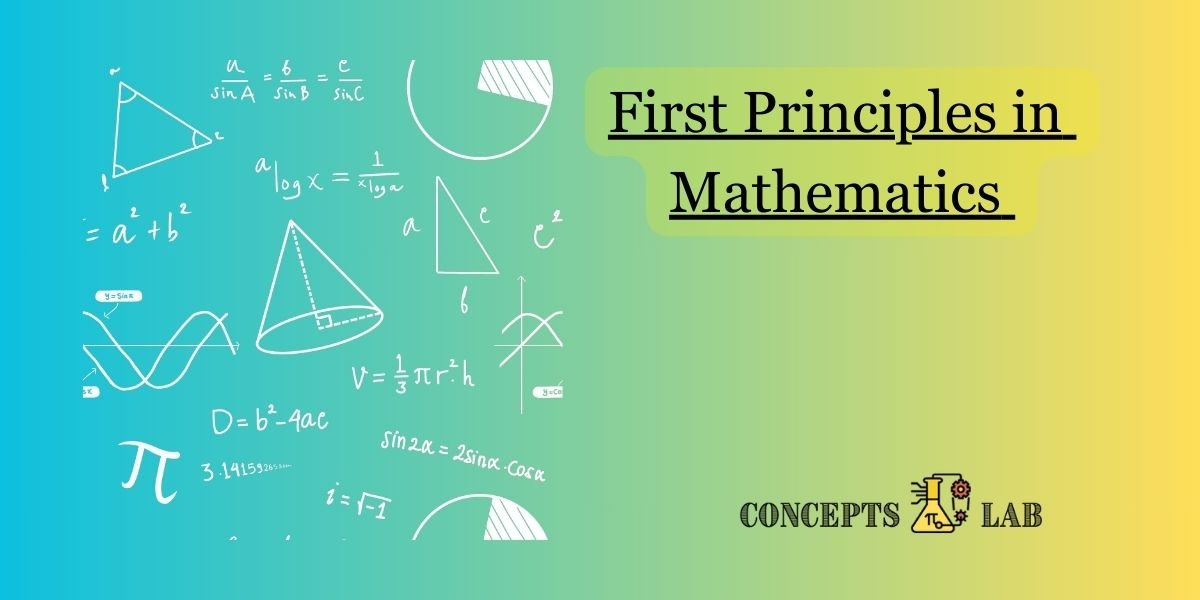In mathematics, “first principles” typically refer to fundamental concepts or axioms that are considered self-evident and are not derived from other principles. These principles serve as the foundation for mathematical reasoning and the development of more complex mathematical structures. Here are some key first principles in mathematics:

1. Axioms of Arithmetic:
• Associative Property: (a + b) + c = a + (b + c)
• Commutative Property: a + b = b + a
• Distributive Property: a(b + c) = ab + ac
2. Real Number Properties:
• Transitive Property of Inequality: If a < b and b < c, then a < c.
• Reflexive Property of Equality: a = a
• Symmetric Property of Equality: If a = b, then b = a
3. Fundamental Theorem of Arithmetic:
• Every integer greater than 1 is either a prime number or can be factorized uniquely into prime numbers.
4. Basic Properties of Exponents:
• Product of Powers: a^m \cdot a^n = a^{m+n}aman=am+n
• Quotient of Powers: a^m / a^n = a^{m-n}am/an=amn
• Power of a Power: (a^m)^n = a^{mn}(am)n=amn
5. Definition of a Function:
• A function is a relation between a set of inputs (domain) and a set of possible outputs (codomain), such that each input is related to exactly one output.
6. Pythagorean Theorem:
• In a right-angled triangle, the square of the length of the hypotenuse (the side opposite the right angle) is equal to the sum of the squares of the lengths of the other two sides.
7. Fundamental Theorem of Calculus:
• It states the relationship between the derivative and the integral and is fundamental to the branch of mathematics known as calculus.
8. Euclidean Geometry Axioms:
• Parallel Postulate: Through a point not on a given line, there is exactly one line parallel to the given line.
• Angle-Side-Angle (ASA) Congruence Axiom: Two triangles are congruent if they have two angles and the included side equal.
9. Fundamental Theorem of Algebra:
• Every non-constant polynomial has at least one complex root.
10. Peano Axioms (for Natural Numbers):
• A set of axioms that define the natural numbers and their properties, used as a foundation for number theory.

These principles form the building blocks of various mathematical disciplines, providing a solid framework for mathematical reasoning and proof.